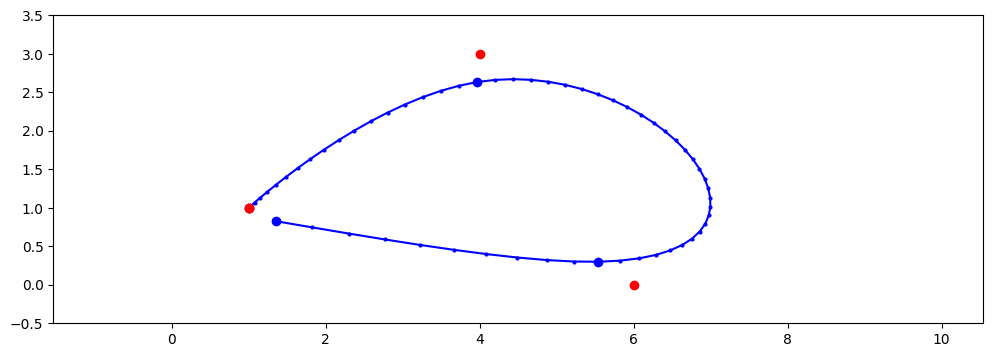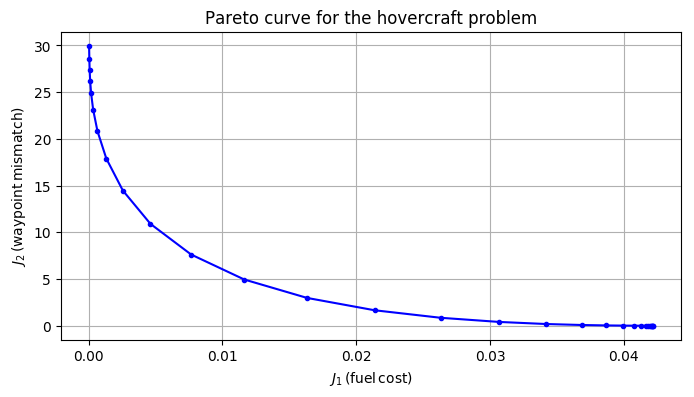# Hovercraft model¶

In :
using JuMP, Gurobi, Mosek

k = 4              # number of waypoints
T = zeros(Int,k)   # vector of timepoints
xw = zeros(2,k)    # vector of waypoint locations

T = 1
T = 20
T = 50
T = 60

# define waypoints
xw = [ 1   4   6   1
1   3   0   1 ]

function solveOpt(λ)
# m = Model(solver = GurobiSolver(OutputFlag=0))
m = Model(solver = MosekSolver(LOG=0))

@variable(m, x[1:2,1:T[k]])  # resulting position
@variable(m, v[1:2,1:T[k]])  # resulting velocity
@variable(m, u[1:2,1:T[k]])  # thruster input

# satisfy the dynamics (with zero initial velocity)
@constraint(m, v[:,1] .== [0;0])

for t in 1:T[k]-1
@constraint(m, x[:,t+1] .== x[:,t] + v[:,t])
@constraint(m, v[:,t+1] .== v[:,t] + u[:,t])
end

#=
# hit all the waypoints
for i in 1:k
@constraint(m, x[:,T[i]] .== xw[:,i])
end
=#

# hit the first waypoint only
for i in 1
@constraint(m, x[:,T[i]] .== xw[:,i])
end

# minimize 2-norm (THIS IS LEAST-SQUARES)
@objective(m, Min, sum(u.^2) + λ*sum( (x[:,T]-xw).^2 ) )
solve(m)

J1 = getvalue(sum(u.^2))
J2 = getvalue(sum( (x[:,T]-xw).^2  ))
xopt = getvalue(x)

return (J1,J2,xopt)
end
;


## Plot trajectory¶

In :
λ = 0.01
(J1x,J2x,x) = solveOpt(λ)

using PyPlot
figure(figsize=(12,4))
plot( x[1,:], x[2,:], "b.-", markersize=4 )
plot( x[1,:][T], x[2,:][T], "b.", markersize=12 )
plot( xw[1,:], xw[2,:], "r.", markersize=12 )
axis("equal")
axis((1.,8.,-.5,3.5));In :
Npts = 30
J1 = zeros(Npts)
J2 = zeros(Npts)
for (i,λ) in enumerate(logspace(-5,1,Npts))
(J1[i],J2[i],uu) = solveOpt(λ)
end;

In :
using PyPlot
figure(figsize=(8,4))
plot( J1, J2, "b.-" )
xlabel(L"J_1\, (\sf fuel\, cost)")
ylabel(L"J_2\, (\sf waypoint\, mismatch)");
title("Pareto curve for the hovercraft problem")
grid()In [ ]: